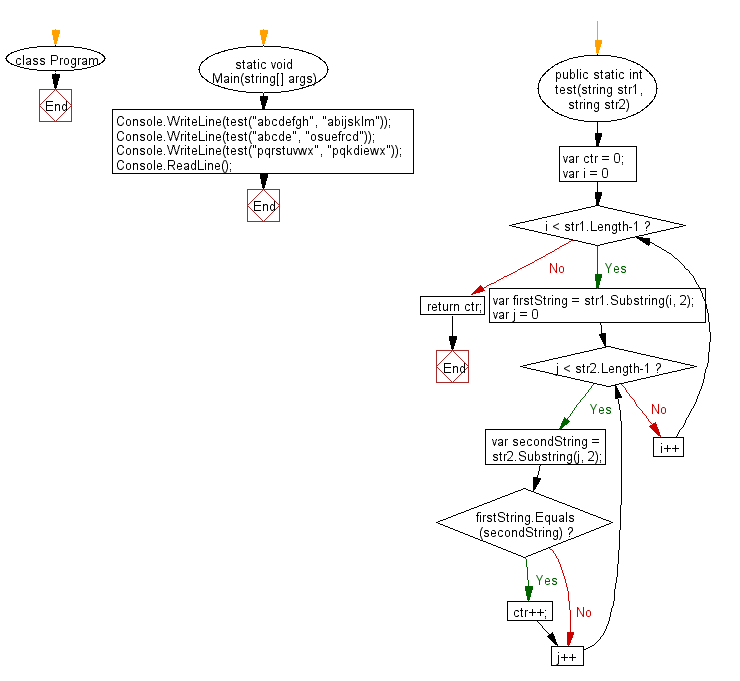﻿ C# - Number of substrings that are the same in two strings# C# Sharp Basic Algorithm Exercises: Compare two given strings and return the number of the positions where they contain the same substring of length 2

## C# Sharp Basic Algorithm: Exercise-35 with Solution

Write a C# Sharp program to compare two given strings and return the number of the positions where they contain the same substring of length 2.

Sample Solution:-

C# Sharp Code:

``````using System;
using System.Linq;

namespace exercises
{
class Program
{
static void Main(string[] args)
{
Console.WriteLine(test("abcdefgh", "abijsklm"));
Console.WriteLine(test("abcde", "osuefrcd"));
Console.WriteLine(test("pqrstuvwx", "pqkdiewx"));
}

public static int test(string str1, string str2)
{
var ctr = 0;
for (var i = 0; i < str1.Length-1; i++)
{
var firstString = str1.Substring(i, 2);
for (var j = 0; j < str2.Length-1; j++)
{
var secondString = str2.Substring(j, 2);
if (firstString.Equals(secondString))
ctr++;
}
}
return ctr;
}
}
}
```
```

Sample Output:

```1
1
2```

Flowchart:C# Sharp Code Editor:

Improve this sample solution and post your code through Disqus

What is the difficulty level of this exercise?

Test your Programming skills with w3resource's quiz.

﻿